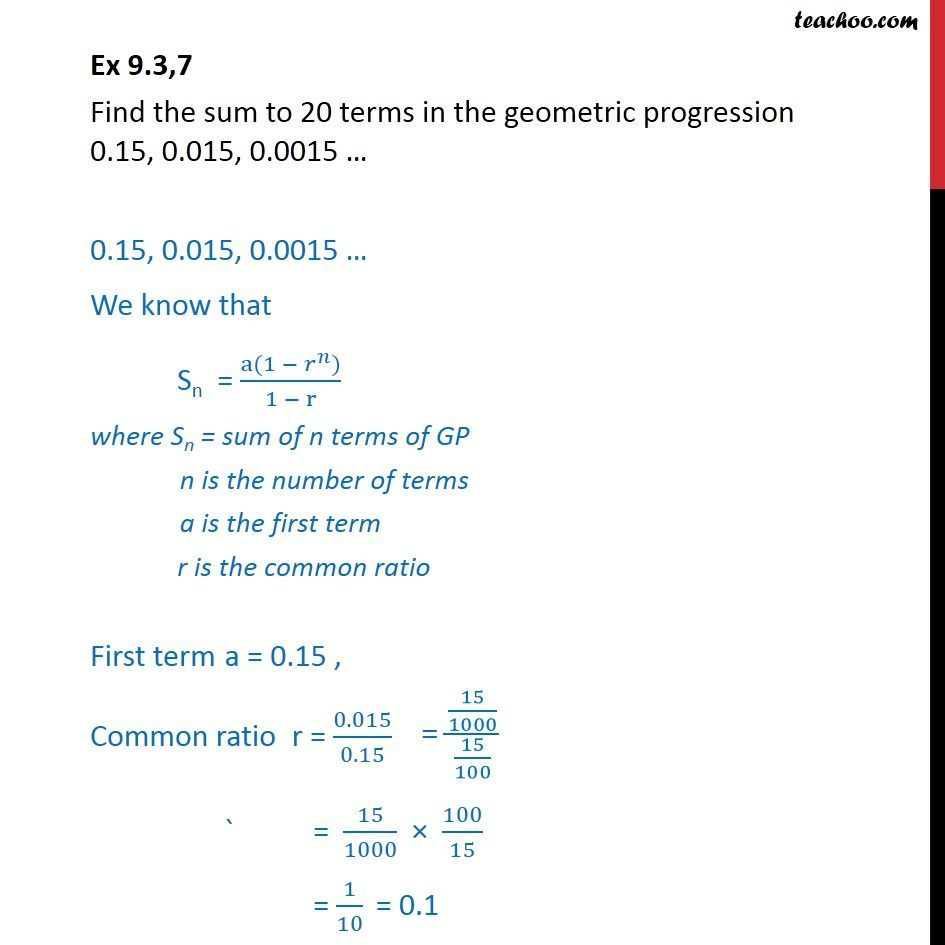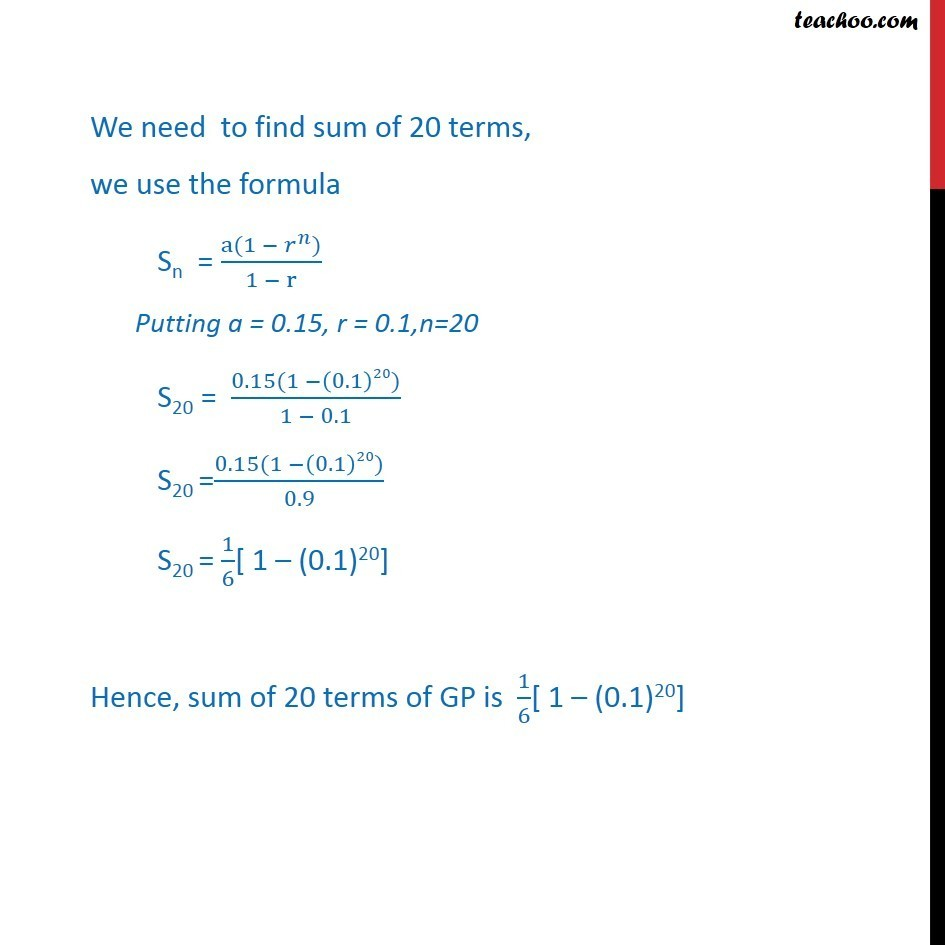Geometric Progression(GP): Formulae based

Chapter 8 Class 11 Sequences and Series
Concept wiseLearn in your speed, with individual attention - Teachoo Maths 1-on-1 Class

### Transcript

Ex 8.2,7 Find the sum to 20 terms in the geometric progression 0.15, 0.015, 0.0015 0.15, 0.015, 0.0015 We know that Sn = (a(1 ^ ))/(1 r) where Sn = sum of n terms of GP n is the number of terms a is the first term r is the common ratio First term a = 0.15 , Common ratio r = 0.015/0.15 ` = 15/1000 100/15 = 1/10 We know that Sum of n term = ( (1 ^ ))/(1 ) We need to find sum of 20 terms. S20 = ( (1 ^20))/(1 ) We need to find sum of 20 terms, we use the formula Sn = (a(1 ^ ))/(1 r) Putting a = 0.15, r = 0.1,n=20 S20 = (0.15(1 (0.1)20))/(1 0.1) S20 =(0.15(1 (0.1)20))/0.9 S20 = 1/6[ 1 (0.1)20] Hence, sum of 20 terms of GP is 1/6[ 1 (0.1)20]